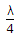# Electronics and Communication Engineering - Microwave Communication

Exercise : Microwave Communication - Section 6
6.
Which of the following is not used as a microwave mixer or detector?
PIN diode
Crystal diode
Schottky barrier diode
Backward diode
Explanation:
No answer description is available. Let's discuss.

7.
Integrated fin line can be utilized as a medium for constructing millimeter wave circuits.
True
False
Explanation:
No answer description is available. Let's discuss.

8.
The secondary constants of a line are
Z0
Z0 and γ
Z0, γ and wave velocity
Z0, γ and L
Explanation:
No answer description is available. Let's discuss.

9.
In a loss less line the propagation constant is equal to
jωLC
LC
jωLC
LC
A rectangular wave guide has two stubs each of lengthconnected at the source end. The stubs are circuited. Then the stubs present